# Parametric Binomial Example - Demonstrate Reliability

This example appears in the Life Data Analysis Reference book.

In this example, we will use the parametric binomial method to design a test to demonstrate a reliability of 90% at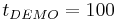${{t}_{DEMO}}=100\,\!$ hours with a 95% confidence if no failure occur during the test. We will assume a Weibull distribution with a shape parameter$\beta =1.5\,\!$.

Determining Units for Available Test Time

In the above scenario, we know that we have the testing facilities available for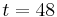$t=48\,\!$ hours. We must now determine the number of units to test for this amount of time with no failures in order to have demonstrated our reliability goal. The first step is to determine the Weibull scale parameter,$\eta \,\!$. The Weibull reliability equation is: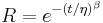$R={{e}^{-{{(t/\eta )}^{\beta }}}}\,\!$

This can be rewritten as: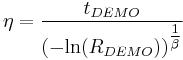$\eta =\frac{{{t}_{DEMO}}}{{{(-\text{ln}({{R}_{DEMO}}))}^{\tfrac{1}{\beta }}}}\,\!$

Since we know the values of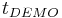${{t}_{DEMO}}\,\!$,${{R}_{DEMO}}\,\!$ and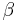$\beta \,\!$, we can substitute these in the equation and solve for$\eta \,\!$: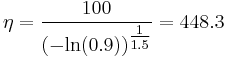$\eta =\frac{100}{{{(-\text{ln}(0.9))}^{\tfrac{1}{1.5}}}}=448.3\,\!$

Next, the value of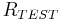${{R}_{TEST}}\,\!$ is calculated by: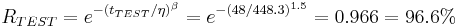${{R}_{TEST}}={{e}^{-{{({{t}_{TEST}}/\eta )}^{\beta }}}}={{e}^{-{{(48/448.3)}^{1.5}}}}=0.966=96.6%\,\!$

The last step is to substitute the appropriate values into the cumulative binomial equation, which for the Weibull distribution appears as: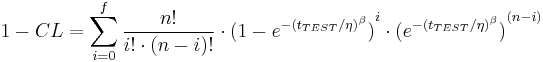$1-CL=\underset{i=0}{\overset{f}{\mathop \sum }}\,\frac{n!}{i!\cdot (n-i)!}\cdot {{(1-{{e}^{-{{({{t}_{TEST}}/\eta )}^{\beta }}}})}^{i}}\cdot {{({{e}^{-{{({{t}_{TEST}}/\eta )}^{\beta }}}})}^{(n-i)}}\,\!$

The values of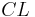$CL\,\!$,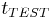${{t}_{TEST}}\,\!$,$\beta \,\!$,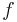$f\,\!$ and$\eta \,\!$ have already been calculated or specified, so it merely remains to solve the equation for$n\,\!$. This value is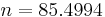$n=85.4994\,\!$, or$n=86\,\!$ units, since the fractional value must be rounded up to the next integer value. This example solved in Weibull++ is shown next.

Determining Time for Available Units

In this case, we will assume that we have 20 units to test,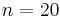$n=20\,\!$, and must determine the test time,${{t}_{TEST}}\,\!$. We have already determined the value of the scale parameter,$\eta \,\!$, in the previous example. Since we know the values of$n\,\!$,$CL\,\!$,$f\,\!$,$\eta \,\!$ and$\beta \,\!$, it remains to solve the binomial equation with the Weibull distribution for${{t}_{TEST}}\,\!$. This value is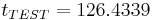${{t}_{TEST}}=126.4339\,\!$ hours. This example solved in Weibull++ is shown next.